# Using MATLAB's ODE solvers

For much of this class, we will use specialized software for understanding the solutions to differential equations, but in the end, you need to be able to run an ODE solver from the command line and plot the results. MATLAB has several such solvers to choose from. For most purposes, the solver ode45 works well.
The graphical tools we have work pretty well for systems of two equations, but for 3D systems become harder to use.
Let's solve the nondimensionalized pendulum equation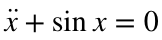which we rewrite as a first order systemA basic call to ode45 has the form
[t,y]=ode45(@f,tspan,y0);
Note that the function must have a header in the exact form dydt=f(t,y). The dependent variable y can be a vector. Here we have replaced the algebraic variables x and y, with the MATLAB variables y(1) and y(2). This program should have a single output dydt of the same dimensions as y.
Now we run the program:
y0=[1 0]; % Initial conditions
tspan=[0 10]; % Time span
[t,y]=ode45(@pendulumODE,tspan,y0);
plot(t,y);legend('x(t)','y(t)');xlabel('t')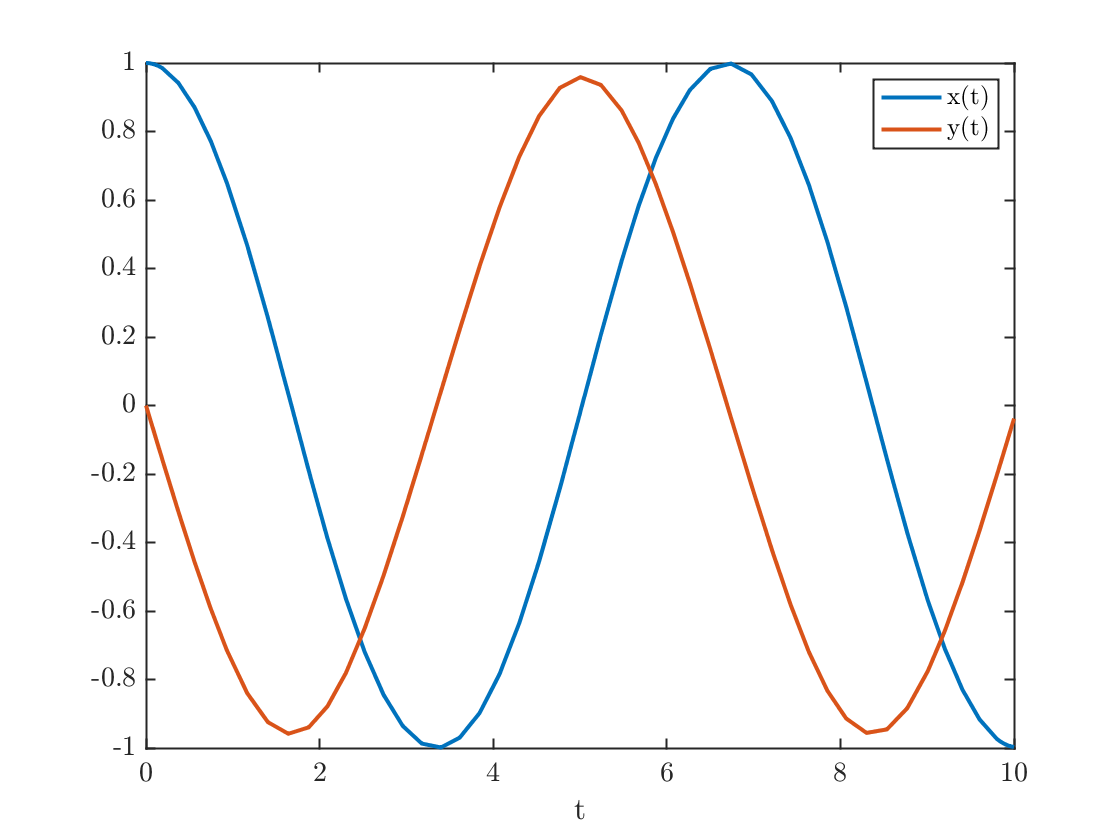figure
plot(y(:,1),y(:,2)); %plot the first column and second column of y as vectors
xlabel('x');ylabel('y');
title('Periodic orbits become closed curves')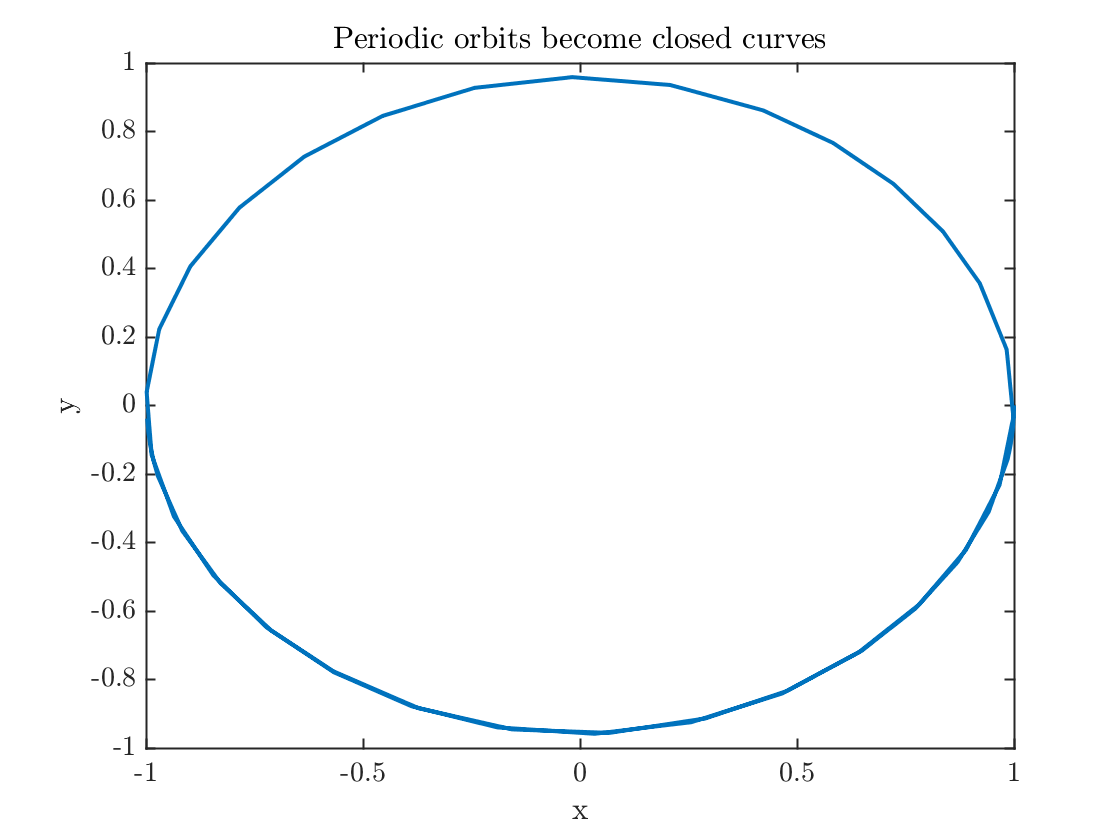#### Inputs

• A function handle. Note that the first input starts with an @ sign. That's because the first input is the name of a function stored in an m-file, not a variable. This is how you tell MATLAB that.
• tspan: This is most simply be a vector containing the start and end times. In this case the output vector t will simply be the times at which the program calculated the solution. If, instead, you want to know the solution at a dicrete sequence of times, then set tspan to be a vector containing all the desired times in order.
• y0: The initial condition vector. Must have the right number of components

#### Outputs

• t: A column vector containing the times at which the solution is found. Let's call its length nt. If tspan has length >2, then t=tspan.
• y: An array whose jth column gives the values of the variable y(j)

## Passing parameters

MATLAB requires that the ODE be defined in a function of the exact form dydt=f(t,y). Many of the ODE we discuss have parameters. We know how to deal with this: anonymous functions. As an example take the glycolysis model on page 207 of Strogatz:This is defined below in the function glycolysis.m. The solution quickly approaches a limit cycle.
a=0.06; b=0.5;
f=@(t,y)glycolysis(t,y,a,b);
tFinal=40;
y0=[b/2,b/a]; % initial condition on top edge figure 7.3.5
[t,y]=ode45(f,[0 tFinal],y0);
figure(1)
plot(t,y);xlabel('$t$');legend('$x$','$y$')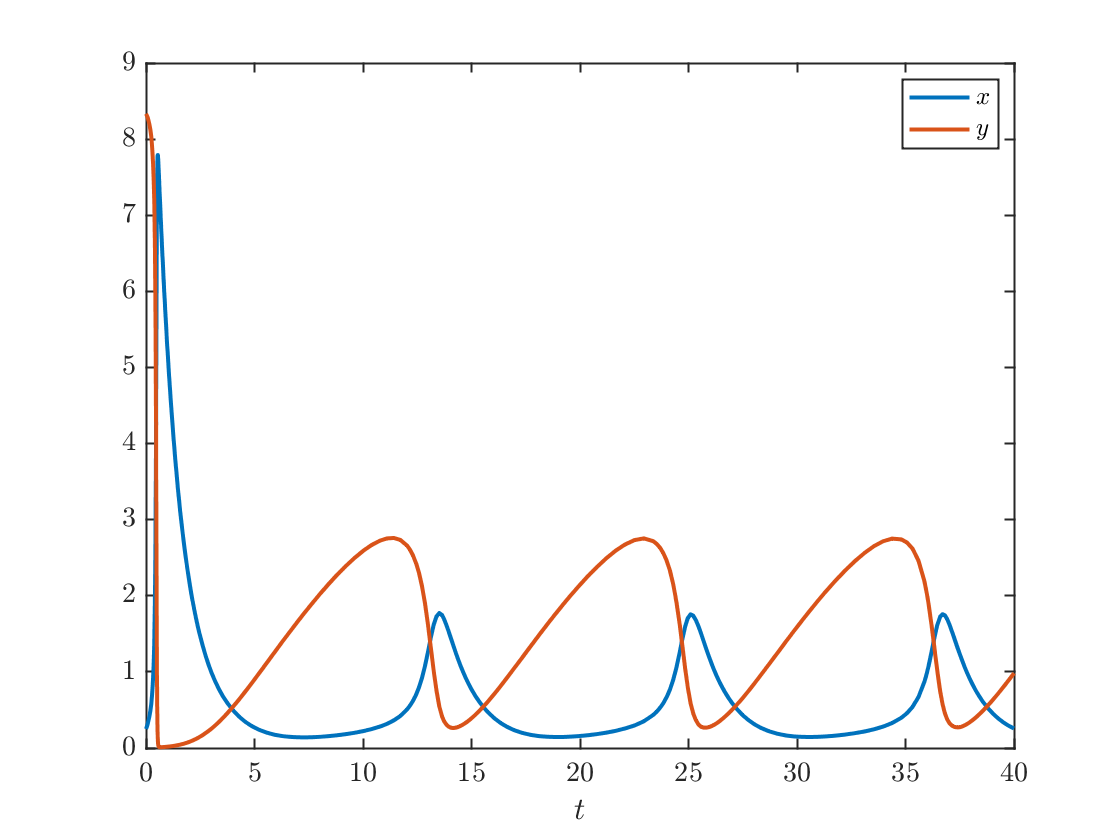figure(2)
plot(y(:,1),y(:,2));xlabel('$x$');ylabel('$y$')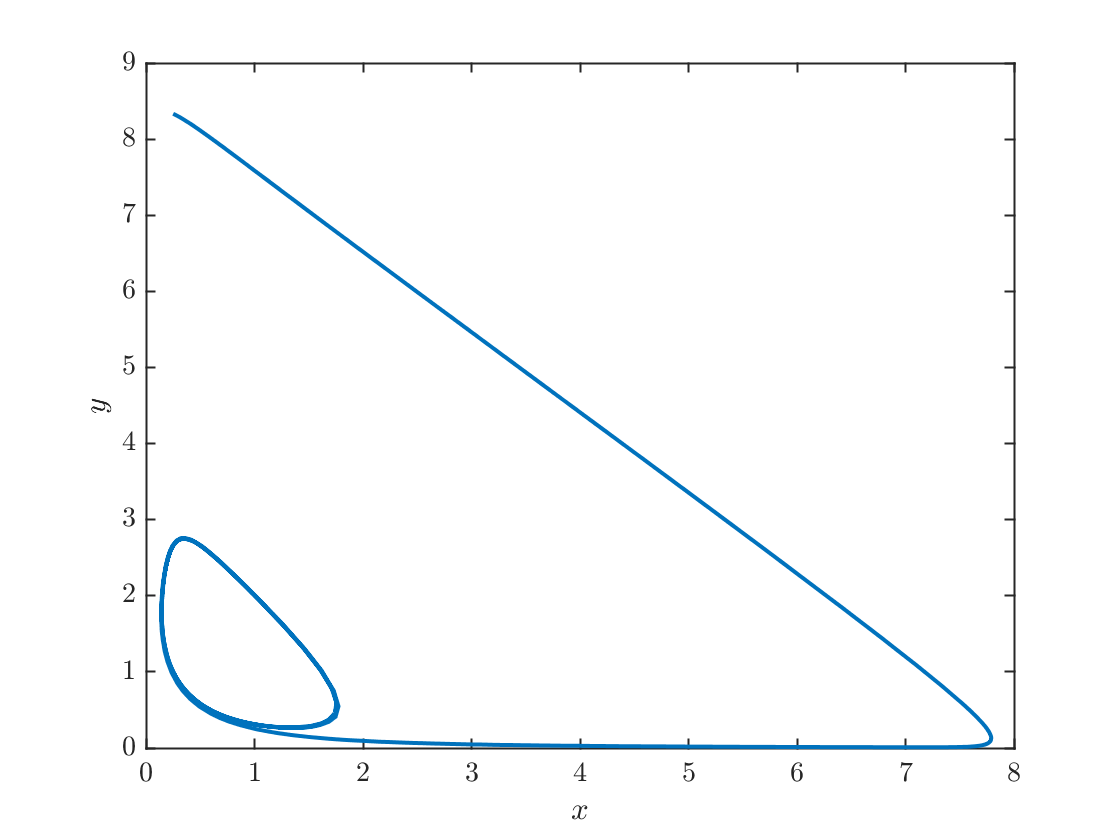## How does ODE45 work?

You all should have learned in your numerical methods class about 4th order Runge-Kutta, a high-order single step method for the numerical solution of ODE. This method evaluates the ODE several times for each time step and combines the outputs to calculate the solution at the next step such that the error scales like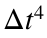. The methods you learned probably use the same value of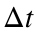throughout the simulation. A smarter thing to do is to use large values ofwhen the solution is changing gradually, but use smaller values ofif the solution is changing more rapidly.
ode45 uses such an adapts the stepsize using the so-called Dormand-Prince method. At time step n it attempts to calculate the next function value using a time step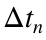. It calculates two approximations: one fourth-order RK approximation and one fifth-order. If the two approximations are sufficiently close, it accepts the fourth order approximation and increases the stepsize slightly for the next step. If the two approximations disagree. It decreasesand tries again. It does this in a clever way so that at each step, it computes five values of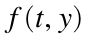and combines them in two ways: one fourth order and one fifth order.
If you inspect the output variable t, you will discover that the time steps vary over the computation. This is a result of the timestep adaptation. Let's see how this looks:
plot(t(1:end-1),diff(t),'.');
xlabel('$t$');ylabel('$\Delta t$')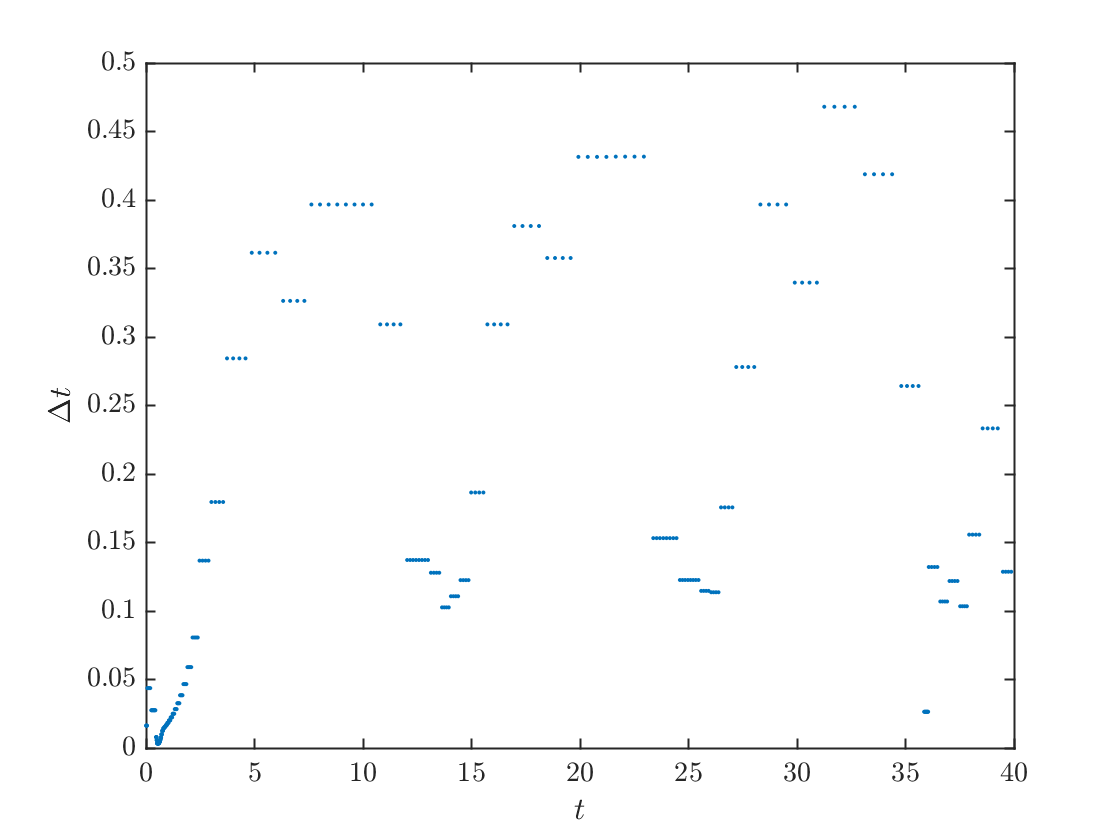fprintf('Minimum stepsize %0.4f\n',min(diff(t)))
Minimum stepsize 0.0033
fprintf('Maximum stepsize %0.2f\n',max(diff(t)))
Maximum stepsize 0.47
Note the minimum stepsize is quite small but that's just what it starts with at the beginning, before it figures out a time step that works better.

## Is ODE45 always the best choice?

It usually works well. If the ODE is stiff, i.e. if it has some large negative eigenvalues, then the stepsizes will get very small and the method will slow down. If the stepsizes get too small it might fail to work altogether. In that case, MATLAB provides an alternate method ode23s that combines two stiff methods, one second order and one third order, that don't suffer from small stepsize restrictions.

1) Consider the system:Write this as a system of equations. Use ode45 to solve this for a variety of initial conditions and plot the trajectories in the phase plane. Describe what you see. You should be able to group the solutions into five families with similar behavior. You may compare your solution with one of the phase plane programs we are using. Bonus: Find a conservation law for this problem and use the MATLAB contour function to draw the level sets.
2) Let's go back to problem 4.4.2 in Strogatz. Numerically solve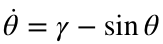for a variety of values of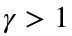. Plot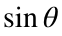as a function of t, and use the time between minima to estimate the period. Try to match the numbers you get to the formula (3) on page 100 of Strogatz, with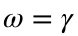and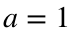.

## Functions called

The pendulum ODE, written as a first-order system
function dydt = pendulumODE(t,y)
dydt=zeros(2,1);
dydt(1)=y(2);
dydt(2)=-sin(y(1));
end
The glycolysis model system, used to demonstrate the use of anonymous functions to pass ODE with parameters to ode45.
function dydt = glycolysis(t,y,a,b)
dydt=zeros(2,1);
dydt(1)=-y(1)+a*y(2)+y(1)^2*y(2);
dydt(2)=b-a*y(2)-y(1)^2*y(2);
end